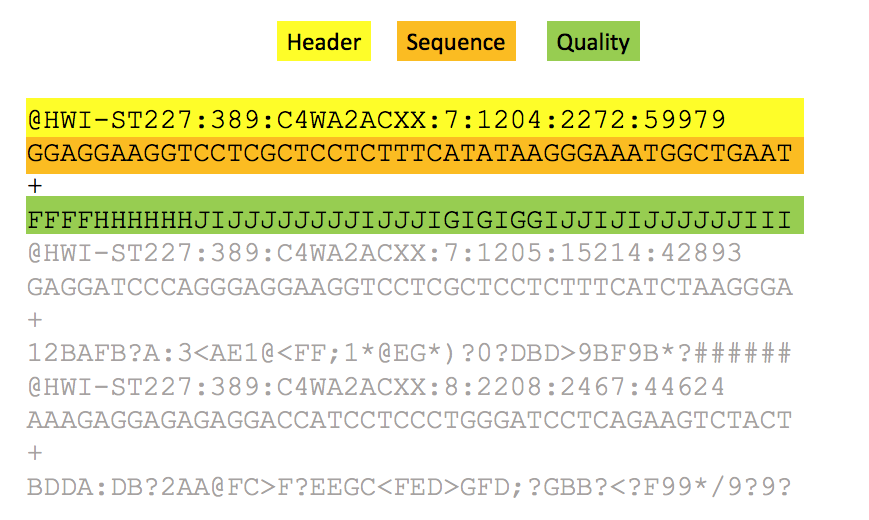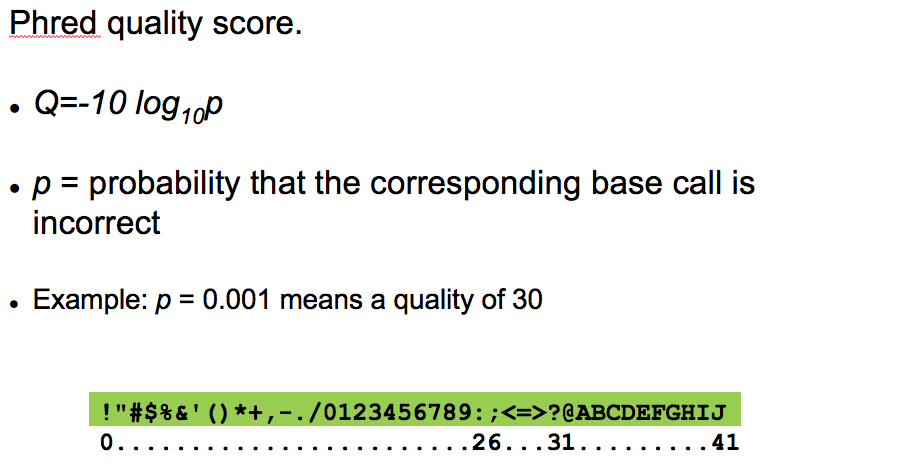# FASTQ format for sequencing reads

Short (and long) sequencing reads coming from the sequencers are stored in FASTQ format (files with an extension .fastq). This format contains the information about the sequence and the quality of each sequenced base. The quality encodes the probability that the corresponding base call is incorrect.The FASTQ format contains four rows per sequencing read:

• a header containing @ as the first character
• the sequence content
• a spacer
• the quality encoded using ASCII characters.• Score = 10 (symbol ‘+’) => probability of incorrect base call = 0.1 => base call accuracy = 90%
• Score = 20 (symbol ‘5’) => probability of incorrect base call = 0.01 => base call accuracy = 99%
• Score = 30 (symbol ‘?’) => probability of incorrect base call = 0.001 => base call accuracy = 99.9% - This is a commonly acceptable threshold for trimming.
• Score = 40 (symbol ‘I’) => probability of incorrect base call = 0.0001 => base call accuracy = 99.99%